# Equivalence of categories

(diff) ← Older revision | Latest revision (diff) | Newer revision → (diff)

An extension of the concept of an isomorphism of categories brought about, first of all, by the presence of classes of isomorphic objects.

Two categoriesand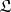are called equivalent if there are one-place covariant functors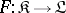and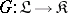such that the productis naturally equivalent to the identity functor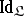and the product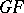to the functor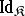; in other words, the categoriesandare equivalent if there are functorsand"almost" inverse to one another. Two categories are equivalent if and only if their skeletons are isomorphic (see Skeleton of a category).

Pontryagin's duality theorem establishes the equivalence of the category of Abelian groups and the category that is dual to that of topological Abelian groups; the category of Boolean algebras is equivalent to the category that is dual to that of Boolean spaces; the category of binary relations over the category of sets is equivalent to the Kleisli category for the triple defined by the functor of taking the set of subsets.